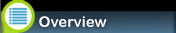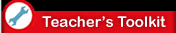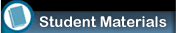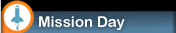Teacher's ToolkitLesson Plans

Lesson Plan 4: Percentages

Introduction
Math problems involving percentages are often presented as “word” problems. Percentages can help make data more digestible. For example, instead of saying that five out of every eight students passed the math test yesterday, you can report that only 62.5 percent of the students passed the test. Students might be able to understand the percentage better. In the M.A.R.S. mission working data into percentages also allows students to make critical decisions about their mission.

Learning to calculate percentages is not difficult. Knowing just a few key terms allows students to easily translate a percentage word problem into a mathematical expression they can solve.

Duration

30 minutes

Materials

• Percentage lesson worksheet

• Pencil

• Calculator (optional)

Procedure

1. Read and review the information on the worksheet in class.

2. Check for understanding throughout the review.

3. Do the problem examples on the board, if necessary, to reinforce the steps for translating the words into mathematical terms.

4. Have the students complete the problems and check for understanding.

How to Translate Percentage Word Problems
Percent” comes from Latin and means "for every hundred.” So, when you hear that “50 percent of the students passed the math test,” it means that for every 100 students, only 50 passed the test.

Of” means “multiplied by.” For example:

What is 50 percent of 20?

What is 50 percent X 20?

“Is” always means “equals.

“Per” means “divided by.” For example:

If you drive 300 miles and you were driving 60 miles per hour, how many hours does your trip take?

300 divided by 60 = 5 hours

“%” means “per one hundred.” To get a decimal that you can use in a multiplication or division problem, divide by 100. For example:

50% means 50 divided by 100, or 0.50

“What” can be replaced by what number you are trying to find. You can usually replace this with “X” to solve the equation you now have in mathematical terms.

The key terms listed above have been summarized in this table. As you review the following examples and work the practice problems, refer to this table to translate the following problems into mathematical expressions you can solve.

 Term Mathematical Expression percent "for every one hundred" of "Multiplied by" or "times" is "equals" per "divided by" % "percent" what (the number you are trying to find can be replaced with "x")

Examples:

1. What is 50 percent of 30?

Translation:  X = .50 × 30

X = 15

1. 11 is what percent of 44?

Translation:  11 = (X/100) × 44?

X = 25 %

Solve these problems:

1. What is 35 percent of 80?

1. 16 percent of what number is 2?

1. 43 percent of what number is 34?

1. Amanda loves blue clothes. She has more blue outfits than any other color of clothes! Her best friend notices that Amanda wears blue for 68 percent of school days. In a school year of 180 days, how many days does Amanda wear Blue?

1. When one of your teachers was 15, he weighed 92 percent of his weight today. Back then he weighed 115 lbs. How much does he weigh today?

1. Michael had a sore throat, and his doctor estimated that he had 156,000 strep bacteria in his throat. By the time he got this medicine, he had 250 percent more bacteria! How many bacteria did he have now?

Solve these problems:

1. What is 35 percent of 80?

x = (35%) (80)
x = (.35) (80)
x = 28

1. 16 percent of what number is 2?

(16) (X) = 2
X = 12.5

1. 43 percent of what number is 34?

(43%) (X) = 32
(.43) (X) = 32
X = 74

1. Amanda loves blue clothes. She has more blue outfits than any other color of clothes! Her best friend notices that Amanda wears blue for 68 percent of school days. In a school year of 180 days, how many days does Amanda wear blue?

(68%) (180) = days
(.68) (180) = 122.4 days

1. When one of your teachers was 15, she weighed 92% of his weight today. Back then she weighed 115 lbs. How much does she weigh today?

(92%) (x) =  115
(.92) (x) = 115
x = 115/.92
x = 125 lbs.

1. Michael had a sore throat, and his doctor estimated that he had 156,000 strep bacteria in his throat. By the time he got this medicine, he had 250 percent more bacteria! How many bacteria did he have now?

Note: 250 divided by 100 = 2.5
(2.5) (156,000) + (156,000) = x
390,000 + 156,000 = x
546,000 bacteria= xAnimation showing all of the innovative programs for digital learning that the Center for Educational Technologies has developed. Some of them include: EVA Alert, M.A.R.S., and Target Moon. The Center for Educational Technologies® at WJU has developed a series of innovative programs for digital learning. These programs provide teachers with an all-inclusive package comprised of curriculum aligned with state and national standards and a live “mission” via technology. The programs were designed around best practice models and principles derived from the latest educational research.